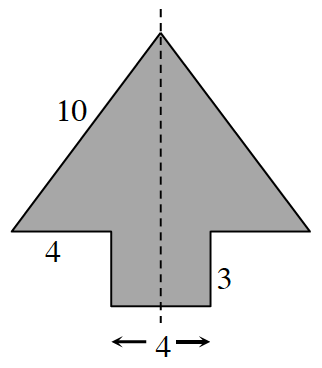### Home > INT2 > Chapter 2 > Lesson 2.1.4 > Problem2-43

2-43.

The dashed line at right represents the line of symmetry of the shaded figure. Calculate the area and perimeter of the shaded region. Show all work.

Because the left and right sides of the figure are identical, the missing sides can be easily filled in to find the perimeter.
To find the area you can divide the figure into a rectangle and a triangle. Use Pythagorean theorem to find the height of the triangle.